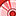# QlikView App Dev

Discussion Board for collaboration related to QlikView App Development.

Announcements
Skip the ticket, Chat with Qlik Support instead for instant assistance.
cancel
Showing results for
Did you mean:Anonymous
Not applicable

## Help needed while calculating a percentage in a text object field

Hello,

I'm trying to calculate a percentage in a text object field.

So far, by using the bellow expression I was able to get the result I want, but it gives me the full % value. But what I really need it a round number with no decimals, or max two decimals numbers.

My values are:

CC: 69

Other: 18

Total (CC + Other) = 87

My expression is:

=count(if([Tipo de contacto]='CC',[Tipo de contacto]))/count([Tipo de contacto])*100 & ' %'

Value gotten so far: 79,310344827586 %

I'm looking for an expression that gives me something like: 79% or 79,31% (with only two decimals)1 Solution

Accepted SolutionsSpecialist III

You can use the Num function to format the number, below should give you 2 decimal places

Num(count(if([Tipo de contacto]='CC',[Tipo de contacto]))/count([Tipo de contacto])*100,'##0.##')

or

no decimal places

Num(count(if([Tipo de contacto]='CC',[Tipo de contacto]))/count([Tipo de contacto])*100,'##0')

https://help.qlik.com/en-US/qlikview/November2017/Subsystems/Client/Content/Scripting/FormattingFunc...

3 RepliesSpecialist III

You can use the Num function to format the number, below should give you 2 decimal places

Num(count(if([Tipo de contacto]='CC',[Tipo de contacto]))/count([Tipo de contacto])*100,'##0.##')

or

no decimal places

Num(count(if([Tipo de contacto]='CC',[Tipo de contacto]))/count([Tipo de contacto])*100,'##0')

https://help.qlik.com/en-US/qlikview/November2017/Subsystems/Client/Content/Scripting/FormattingFunc...Anonymous
Not applicable
Author

It is correct, it works:

=Num(count(if([Tipo de contacto]='CC',[Tipo de contacto]))/count([Tipo de contacto])*100,'##0,##')& ' %'

This gives the result I want. Many thanks!MVP

Also, suggest you to look into set analysis rather than using if statement

=Num(Count({<[Tipo de contacto] = {'CC'}>} [Tipo de contacto])/Count([Tipo de contacto]), '#0.0%')

or this if you want it to be 0 when CC is not selected in Tipo de contacto

=Num(Count({<[Tipo de contacto] *= {'CC'}>} [Tipo de contacto])/Count([Tipo de contacto]), '#0.0%')Community Browser